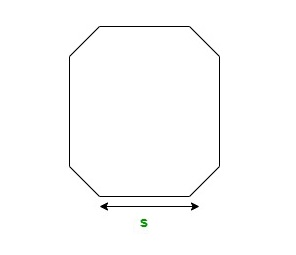GeeksforGeeks App
Open AppBrowser
Continue

## Related Articles

Geometry is a mathematical branch that is about the study of shapes. The category of shapes is divided into two viz. flat shapes and solid shapes. Geometry deals with the study of the area, perimeter, volume, and other parameters of these shapes by giving standard formulas.

The article explains the octagon formula which gives the formula of area and perimeter of an octagon. It also comprises sample numerical problems for better understanding.

### Octagon

Octagon is a plane shape having eight sides and eight angles. It is a regular polygon of eight sides. Each interior angle of the octagon measures 135° and the sum of all the interior angles of an octagon equals 108°. Similarly, the exterior angle of an octagon is 45 degrees and the sum of all the exterior angles equals 360°.

Octagon consists of 20 diagonals that meet at the center of the figure. All these diagonals have the same length.Regular octagon

### Octagon Formula

The geometry provides separately derived formulas for the calculation of perimeter, area, and diagonals of a regular octagon. The perimeter, area, and diagonal formula of an octagon is collectively known as the Octagon Formula.

To find the number of diagonals of an octagon we use the given formula.

Number of Diagonals = n(n – 3)/2

8(8 – 3)/2

20

Where s denotes  side length

And, n denotes the number of sides

A regular polygon generally consists of 20 diagonals. So, the octagon formula is mostly used to calculate the area and perimeter of an octagon. These calculations are carried out by using the length of a side of the octagon.

The area formula of  an octagon is given by

Area of octagon(A) = 2s2(1 + √2)

Where s is the length of a side

The perimeter formula of an octagon is given by,

The perimeter of the octagon(P) = 8s

Where s is the length of a side.

### Sample Problems

Question 1: Find the area and perimeter of an octagon having a side 2cm using the octagon formula.

Solution:

Given:

length of a side of the octagon is 2cm

By using the octagon formula for the area

Area of octagon(A) = 2s2(1 + √2)

A = 2(2)2(1 + √2)

A = 19.31cm2

By using the octagon formula for the perimeter,

Perimeter of the octagon (P) = 8s

P = 8 × 2

P = 16cm

Hence, the area and perimeter of the given octagon are 19.31cm2 and 16cm respectively.

Question 2: Find the area and perimeter of an octagon having a side of 4cm using the octagon formula.

Solution:

Given:

length of a side of the octagon is 4cm

By using the octagon formula for the area

Area of octagon(A) = 2s2(1 + √2)

A = 2(4)2(1 + √2)

A = 77.25cm2

By using the octagon formula for the perimeter

The perimeter of the octagon (P) = 8s

P = 8 × 4

P = 32cm

Hence, the area and perimeter of the given octagon are 77.25cm2 and 32cm respectively.

Question 3: Find the area and perimeter of an octagon having a side of 2.5cm using the octagon formula.

Solution:

Given:

length of a side of the octagon is 2.5cm

By using the octagon formula for the area

Area of octagon(A) = 2s2(1 + √2)

A = 2(2.5)2(1 + √2)

A = 30.17cm2

By using the octagon formula for the perimeter

The perimeter of the octagon (P)=8s

P = 8 × 2.5

P = 20cm

Hence, the area and perimeter of the given octagon are 30.17cm2 and 20cm respectively.

Question 4: A regular octagon is given which has a perimeter equal to 32cm. Find its area using the octagon formula.

Solution:

Given:

The perimeter of the octagon is 32cm.

The perimeter of the octagon(P) = 8s

32 = 8s

s = 4cm

By using the octagon formula for the area

Area of octagon(A) = 2s2(1 + √2)

A = 2(4)2(1 + √2)

A = 77.25cm2

Question 5: A regular octagon is given which has a perimeter of 48cm. Find its area using the octagon formula.

Solution:

Given:

The perimeter of the octagon is 48cm.

The perimeter of the octagon(P) = 8s

48 = 8s

s = 6cm

By using the octagon formula for the area

Area of octagon(A) = 2s2(1 + √2)

A = 2(6)2(1 + √2)

A = 173.82cm2

Question 6: If the perimeter of an octagon is given which is equal to 40cm. Calculate the area of the given octagon.

Solution:

Given:

The perimeter of the octagon is 40cm.

The perimeter of the octagon(P) = 8s

40 = 8s

s = 5cm

By using the octagon formula for the area

Area of octagon(A) = 2s2(1 + √2)

A = 2(5)2(1 + √2)

A = 120.71cm2

Question 7: If an octagon is given having length of 3cm, its area and perimeter be, calculated using the octagon formula?

Solution:

Given:

The side of the octagon is 3cm

By using the octagon formula for the area

Area of octagon(A) = 2s2(1 + √2)

A = 2(3)2(1 + √2)

A = 43.45cm2

By using the octagon formula for the perimeter

The perimeter of the octagon (P) = 8s

P = 8 × 3

P = 24cm

Hence, the area and perimeter of the given octagon are 43.45cm2 and 24cm respectively.

My Personal Notes arrow_drop_up
Related Tutorials